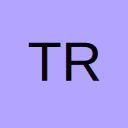# Rank if and statement

• The following is a formula i am using,

=IF(D9="","",RANK(D9,\$D\$9:\$F\$9))

this Ranking formula is working for me fine but what i am trying to do is if is possible to have an if, and, formula then rank.
so in words it would only Rank IF =(D\$3+D\$4+D\$5)>=\$B\$4 AND =(D\$6+D\$7)>\$B\$7 AND =D\$8>=\$B\$8 otherwise FAIL and then to copy this over the other ranking cells to also incorporate the IF(D9="","") part as i will have empty cells within the rank selection.

I have attached also my book for reference.

## Files

• Re: Rank if and statement

So what would a sample answer look like (and why)

Regards

Ford

• Re: Rank if and statement

hope this is what you wanted:

=IF(D9="","",IF(AND((D3+D4+D5)>=B4,(D6+D7)>B7,D8>=B8),RANK(D9,\$D\$9:\$F\$9),"FAIL"))

• Re: Rank if and statement

Quote from MrRedli;768333

hope this is what you wanted:

=IF(D9="","",IF(AND((D3+D4+D5)>=B4,(D6+D7)>B7,D8>=B8),RANK(D9,\$D\$9:\$F\$9),"FAIL"))

MrRedli

This is somewhat of what i am looking for, but the above statement doesnt seem to be ranking 1-5 if it meets the conditions -
D3+D4+D5)>=B4,(D6+D7)>B7,D8>=B8)

what is happening is its just putting in FAIL into all where there should be a rank number even when it meet the condition.

Only if it meets the condition do i want to rant 1-5 if not i want the FAIL.

• Re: Rank if and statement

hi Trish, on what bases is the ranking? this is what I had thought you are trying to do...

## Files

• Re: Rank if and statement

ive expanded on the initial table i was working on ans also the query as follows

=IF(F18="","",IF(AND((F12+F13+F14)>=\$D\$13,(F15+F16)>=\$D\$16,F17>=\$D\$17),RANK(E19,\$E\$19:\$AT\$19),"FAIL"))

At the moment when the conditions are met the above query is not ranking form me it just seems to be not working correctly it doesn't even seem to be ranking correctly, i have attached the updated book, its probably something i did incorrect and mixed it up.

forum.ozgrid.com/index.php?attachment/68773/

## Files

• Re: Rank if and statement

update the formula as below:

=IF(F18="","",IF(AND((F12+F13+F14)>=\$D\$13,(F15+F16)>=\$D\$16,F17>=\$D\$17),RANK(F18,\$E\$18:\$AT\$18),"FAIL"))

• Re: Rank if and statement

MrRedi,

Thank you this is working perfectly, and i see what i was doing incorrectly in the Rank. :thanx:

## Participate now!

Don’t have an account yet? Register yourself now and be a part of our community!